Science Journal of Applied Mathematics and Statistics
Volume 3, Issue 3, June 2015, Pages: 160-164

Reliability Equivalence Analysis of a Parallel-Series System Subject to Degradation Facility

M. A. El-Damcese

Department of Mathematics, Faculty of Science, Tanta University, Tanta, Egypt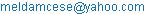M. A. El-Damcese. Reliability Equivalence Analysis of a Parallel-Series System Subject to Degradation Facility. Science Journal of Applied Mathematics and Statistics. Vol. 3, No. 3, 2015, pp. 160-164. doi: 10.11648/j.sjams.20150303.19

Abstract: The performance of a reliability system can be improved by different methods, e.g. the reliability of one or more components can be improved, hot or cold redundant components can be added to the system. Sometimes these measures can be equivalent as they will have the same effect on some performance measure of the system. This paper discusses the reliability equivalences of a parallel–series system. The system considered here consists of m subsystems connected in parallel, with subsystem i consisting of ni independent and identical components in series for i=1, 2, …, m. Three different methods are used to improve the system reliability: (i) the reduction method, (ii) the hot duplication method and (iii) the cold duplication method. Each component of the system has four states and two types of partial failure rates. In this study, the lifetimes of the system components are exponentially distributed. A numerical example is introduced to illustrate how the idea of this work can be applied.

Keywords: Partial Failure Rate, Reliability Equivalence Factors, Parallel-Series System

1. Introduction

In reliability theory, one way to improve the performance of a system is to use the redundancy method. There are two main such methods:

1. Hot duplication method: in this case, it is assumed that some of the system components are duplicated in parallel.

2. Cold duplication method: in this case, it is assumed that some of the system components are duplicated in parallel via a perfect switch.

Unfortunately, for many different reasons, such as space limitation, high cost, etc, it is not always possible to improve a system by duplicating some or all of its components. For example, satellites and space aircrafts have limited space which doesn't allow component duplication. Also, some microchips are so expansive that manufacturers cannot afford to duplicate them. In such cases where duplication is not possible, the engineer turns to another well-known method in reliability theory, the so-called reduction method. In this method, it is assumed that the failure rates of some of the system components are reduced by a factor ρ, 0 < ρ < 1. Now, once the reduction method is adopted, the main problem facing the engineer is to decide to what degree the failure rate should be decreased in order to improve the system. To solve this problem, one can make equivalence between the reduction method and the duplication method based on some reliability measures. In other words, the design of the system improved by the reduction method should be equivalent to the design of the system improved by one of the duplication methods. The comparison of the designs produces the so-called reliability equivalence factors by Sarhan et al. (2008). The concept of the reliability equivalence factors was introduced in the report Rade (1989-1) and applied to various reliability systems by Rade (1989-3, 1991). Rade (1993a, 1993b) applied this concept for the two-component parallel and series systems with independent and identical components whose lifetimes follow the exponential distribution. Xia and Zhang (2007) considered equivalence factors in Gamma distribution. El-Damcese and Khalifa (2008) obtained the reliability equivalence factors of series-parallel systems in the Weibull distribution. Mustafa and El-Faheem (2012) found the reliability equivalence factors of a general parallel system with mixture of life time distributions.

Also, Shawky et al. (2013) considered the reliability equivalence for the exponentiated exponential distribution. In the previous mentioned studies, the hazard and the reliability functions are decreases or increases through the indexed scale parameter. In reliability general frame analysis, there exists other lifetime distributions for which the hazard and reliability functions are not affected by the scale parameter, and mainly affected by the shape parameter. Burr type X distribution initially proposed by Burr (1942) and investigated a generalization of the Rayleigh distribution by Mudholkar and Srivastava (1993). This distributions effectively modeled in general lifetime data and considered by Migdadi and Al-Batah (2014). Abdelfattah and El-Faheem (2014) applied generalize reliability equivalence technique to apply it to a system of mixture of n independent and non-identical lifetimes with delay time.

The structure of this paper is organized as follows. Section 2 presents the reliability of component subject to four states and two types of partial failure rates. Section 3 introduces the illustration of the parallel-series system and the system reliability. Section 4 presents the reliability of the systems improved according to three different methods that can be applied to improve the performance of the original system. In Section 5, two types of reliability equivalence factors of the system are discussed. A numerical example is introduced in Section 6 to illustrate how the idea of this work can be applied. Finally, Section 7 is devoted to the conclusion.

2. Reliability of Component

Considering the component with two types of partial failure rates. The Markov method is used to develop generalized expressions for component state probabilities; component reliability. According to the model assumptions, when the lifetime of component is assumed to be exponentially distributed, then the state of the component at time t {𝑁(𝑡), 𝑡 0} is a homogeneous continuous-time Markov chain with state space Ω = {3, 2,1, 0}. The set of working/ degraded states is given by 𝑊 = {3, 2, 1}, and the set of failure states is given by F= {0}.

The initial conditions for this problem are: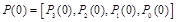(1)

The differential equations of the (working /degraded) state probabilities written in the matrix form are given by: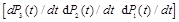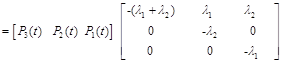(2)

where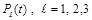probability that the component is in (degraded /working) state at time t,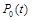probability that the component is in down state at time t,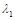the failure rate of a component when it goes from up state to degraded state of type 1,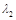the failure rate of a component when it goes from up state to degraded state of type 2.

Using the initial condition from equation (1) and obtain the values of required state probabilitiesfrom equation (2) which are: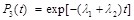,

and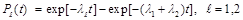The component reliability function at time t is: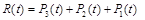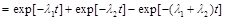(3)

3. Parallel –Series System

We suppose that the system consists of m subsystems connected in parallel and each subsystem consists of ni components connected in series for i = 1, 2, …, m. The system operates successfully when at least one of its subsystems is up and each subsystem works successfully when all components are up (see Figure 1). We consider that the components of each subsystem are independent and identical. The failure rates of each component are constant. Let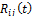be the reliability function of the component j ( j = 1, 2, …, ni) in subsystem i (i = 1, 2, …, m) and let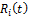be the reliability function of the subsystem i. Hence, the reliability function of the original system is given by: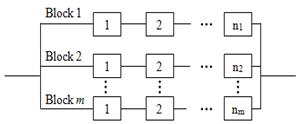Figure 1. Parallel-series system structure.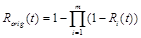(4)

Assuming that, the system components are independent and identical having the failure rates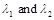, this implies that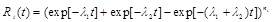(5)

Using (4) and (5), the reliability function of the original system will take the form: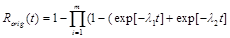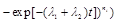(6)

Using equation (6), the mean time to system failure (MTTSForig) can be derived in the following form: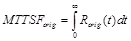(7)

4. The Improved Systems

The reliability of the system can be improved according to one of the following two different methods:

1- Reduction method.

2- Standby redundancy method:

(a) Hot standby redundancy, called hot duplication method

(b) Cold standby redundancy, called cold duplication method.

In the following sections, we will derive the reliability functions and the mean time to failures of the systems improved according to the methods mentioned above.

4.1. The Reduction Method

In this method, it is assumed that the reliability of kini identical components of the subsystem i, i=1, 2, .., m is improved by increasing the reliability function through multiplying the failure rates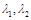by factors ρ and s respectively , 0 < ρ, s <1. Therefore, using (3), the reliability of each of the ki components of the subsystem i, i=1, 2, .., m is given by: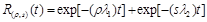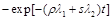(8)

This implies, the reliability of the system improved by the reduction method is given by: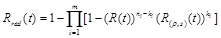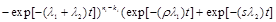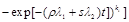(9)

Using equation (9), the mean time to system failure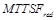can be derived in the following form: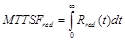(10)

4.2. Hot Duplication Method

In this method, it is assumed that some of the system components are duplicated in parallel. If i, i=1, 2, …, m components are hot duplication, the reliability for each of the i, i=1, 2, …, m components is given by:(11)

This implies, the reliability of the system improved by the hot duplication method is given by: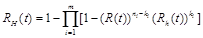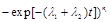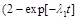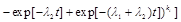(12)

Using equation (12), the mean time to system failure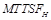can be derived in the following form: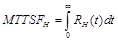(13)

4.3. Cold Duplication Method

In this method, some of the system components are duplicated in parallel via a perfect switch. Following Rade (1989-1), the reliability function of each component improved by a cold via perfect switch can be given by: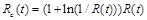(14)

This implies, the reliability of the system improved by the cold duplication method is given by: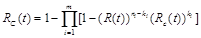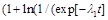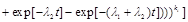(15)

Using equation (15), the mean time to system failure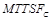can be derived in the following form: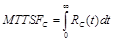(16)

5. Reliability Equivalence Factors

A reliability equivalence factor is a factor by which a characteristic of components of a system design has to be multiplied in order to reach equality of a characteristic of this design and a different design regarded as a standard (Migdadi and Al-Batah (2014)).

As mention above, the reliability equivalence factor is defined as the factor by which the failure rates of some of the system’s components should be reduced in order to reach equality of the reliability of another better system.

In this section, the reliability equivalence factors of the improved systems are derived. The reliability equivalence factor(s) denoted by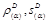, D = H, (C) for hot, (cold) duplication is defined as that factor(s)by which the failure rates for the set of system components should be reduced, or equivalently the reliability function increased so that one could obtain a design of the system with a reliability function of a design obtained from the original system.

For the hot duplication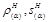can be obtained by solving the set of the two equations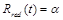and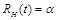(17)

For the cold duplication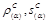can be obtained by solving the set of the two equationsand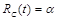(18)

6. Illustrative Example

In this example, we consider that the parallel-series system is consisted of two subsystems connected in parallel (m = 2) and consider that the first subsystem has three components in series (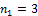) and the second one has two components in series (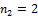). Our aim is to improve the reliability of this parallel-series system by improving the performance of some components instead of increasing the number of these components.

The functions of reliability of the system (for k1= k2 =1) are defined as follows:,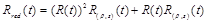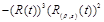,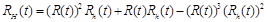,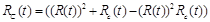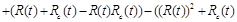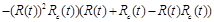.

where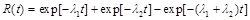For example, given that λ1 =0.001, λ2 =0.003, ρ=0.3 and s=0.5, one may be tempted to calculate the functions of reliability of the system versus the time are shown in Figure 2.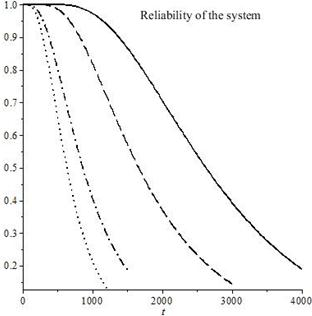Figure 2. The RC(t) (solid line), RH(t) (dashed line), Rred(t) (dashed dotted line), Rorig(t) (dotted line).

Also calculated the mean time to system failure and the results are shown in Table 1.

Table 1. The mean time to failure of the original and improved systems.

 MTTSF MTTSForig MTTSFred MTTSFH MTTSFC Value 739.544 1038.526 1924.505 2637.315

Table 2 represent the α-fractiles, the hot duplicationand cold duplicationcorresponding to RH(t) and RC(t) for k1= k2 =1, when Rred(t) for k1=0, k2 =2 can be derived as follows: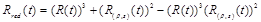and in these calculation the level is chosen to be different values of α-fractiles.

Table 2. The α-fractiles,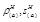,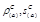.

 α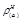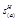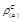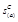0.303 0.343 0.280 0.328 0.110 0.409 0.241 0.820 0.365 0.090 0.504 0.213 0.900 0.335 0.088 0.571 0.215 0.512 0.289 0.932 0.703 0.180 0.498 0.255 0.086 0.819 0.162 0.389 0.240 0.070 0.906 0.150 0.280 0.175 0.067 0.999 0.010 0.020 0.087 0.009

7. Conclusion

In this paper we discussed the reliability equivalence of a parallel-series system with independent and identical components. It is assumed that the each component of the system having two types of partial failure rates. Three ways namely the reduction, hot duplication and cold duplication methods are used to improve the system reliability. A reliability equivalence factor was derived. A numerical example is used to illustrate how the results obtained can be applied.

References

1. Abdelfattah, M.andEl-FaheemAdel A.( 2014), Reliability equivalence factors of a system with mixture of n independent and non-identical lifetimes with delay time, Journal of the Egyptian Mathematical Society, 22, 96–101.
2. Burr, I. W. (1942), Cumulative frequency functions, The Annals of Mathematical Statistics, 13(2), 215-222.
3. El-Damcese, M. A. and Khalifa M. M.(2008),Reliability equivalence factors of a series-parallel systems in Weibull distribution.International Journal of Reliability and Applications, 9(2), 153-165.
4. Migdadi, H. S. and Al-Batah, M. S.( 2014), Testing Reliability Equivalence Factors of a Series- Parallel Systems in Burr Type X Distribution, British Journal of Mathematics & Computer Science, 4(18), 2618-2629.
5. Mudholkar G.S., Srivastava D.K. (1993), Exponentiated Weibull family for analyzing bathtub failure ratedata, IEEE Transactions on Reliability, 42(2),299-302.
6. Mustafa, A. and El-Faheem, A. A.( 2012), Reliability equivalence factors of a general parallel system with mixture of lifetimes. Applied Mathematical Sciences, 6(76), 3769-3784.
7. Rade, L., Reliability Equivalence, Studies in Statistical Quality Control and Reliability 1989-1, Mathematical Statistics, Chalmers University of Technology.
8. Rade, L., Reliability Systems of 3-state Components, Studies in Statistical Quality Control and Reliability 1990-3, Mathematical Statistics, Chalmers University of Technology.
9. Rade, L. Performance Measures for Reliability Systems with a Cold Standby with a Random Switch, Studies in Statistical Quality Control and Reliability 1991 , Chalmers University of Technology.
10. Rade, L. (1993a), Reliability Equivalence, Microelectronics & Reliability, 33(3), 323-325.
11. Rade, L. (1993b), Reliability Survival Equivalence, Microelectronics & Reliability, 33(6), 881-894.
12. Sarhan, A. M., Tadj, L.,Al-khedhairi, A.and Mustafa, A. (2008), Equivalence Factors of a Parallel-Series System, Applied Sciences, 10, 219-230.
13. Shawky, A. I., Abdelkader, Y. H. and Al-Ohally, M. I.( 2013), Reliability equivalence factors in exponentiated exponential distribution. Wulfenia Journal, 20(3), 75-85.
14. Xia, Y. and Zhang, G.( 2007), Reliability equivalence factors in Gamma distribution. Applied Mathematics and Computation, 187(2), 567-573.

 Contents 1. 2. 3. 4. 4.1. 4.2. 4.3. 5. 6. 7.
Article ToolsAbstractPDF(384K)